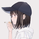7142瀏覽
A detrended series that oscilates around zero is obtained after first differencing a time series (i.e. subtracting the closing price for a candle from the one immediately before, for example). Hypothetically, assuming that every detrended closing price is independent of each other (what might not be true!), these values will follow a normal distribution with mean zero and unknown variance sigma squared (assuming equal variance, what is also probably not true as volatility changes over time for different pairs). After studentizing, they follow a Student's t-distribution, but as the sample size increases (back periods > 30, at least), they follow a standard normal distribution.

This script was developed for personal use and the idea is spotting candles that are at least 99% bigger than average (using N = 3) as they will cross the upper and lower confidence interval limits. N = 2 would roughly provide a 95% confidence interval.

I recommend the standard values N = 2 and N = 3 that provide, respectively, approximately 95% and 99% confidence intervals.
Note: I suggest using smaller sample sizes (between the 30 and 100 last candles) for sigma estimation as they tend to represent better the recent volatility. I also suggest to use sample size=400 for long-term average volatility.

Remark: the original interpretation is a bit misleading. When the series crosses over the interval limits, one can say that the current candle length is 95% or 99% as extreme as the expected length.
Also in hypothesis testing, one could say that the hypothesis of the candle length being within the expected range is rejected at 5% or 1% significance level.

As closing prices can be seen as a random walk on chart, this series is basically modelling its error.

An analogous approach for candle length is just thinking of it as changes in the closing price ( I would rename ir as Price Change Outlier Detector if TV allowed it!).
A green column means a positive change in price and a red column means a negative change in price. These changes are always relative to the last price.

If a column crosses from from the inner band to the outer band, the change in price is considered to be approximately 95% as extreme as expected.
If it crosses both bands, the change in price it considered to be approximately 99% as extreme as expected.

## 評論kiero
@kiero, can be used along with other indicators (RSI and Volume, for example) to detect dips as there's always a fast change in price. Candles that are 99% longer than expected can be good indicators. Of course everybody knows when a candle is locally longer than average, so this oscillator is just one formal way to measure if they lie within the "expected" range.Personally I found that would be more helpful for identifying long wick candles, namely flash crashes, with the "source" of "hl2", but not "close" the default. I guess it would work more nicely with RSI extremes or bollinger extremes or likes. Anyways, nice script! Thanks.fumiyaorg
@fumiyaorg, I didn't know bollinger bands before writing this one, but the idea is almost the same. The only difference is that in a detrended series, this oscillator measures whether each candle (or simply, the change in price from the last closing) is longer than what is "expected". Imo, in bollinger bands the idea is more to check wheter a local trend is within the expected range, as it usually crosses the confidence bands after many candles deviate from the moving average.